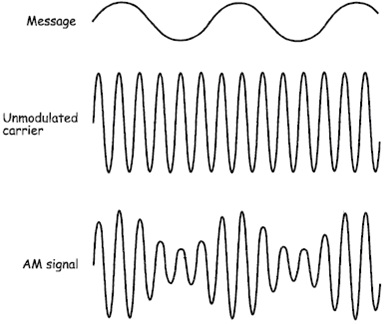Amplitude Modulation

Objective
1. To understand the characteristics of amplitude modulation ( AM ).
2. To study AM modulation index and its effects on the system performance.

Introduction

Amplitude Modulation is a technique used in electronics communication for transmitting information via a carrier wave. It works by varying the strength of the transmitted signal in relation to the information being sent.

Modulation

Modulation is the process whereby some characteristics of one wave is varied in accordance with characteristics of another wave. In other words we can say that modulation is the process of mixing or addition of information signal with an electronic or optical carrier.

Carrier

The sinusoidal signal that is used in the modulation is known as carrier signal or carrier. A carrier wave or carrier is a waveform that is modulated with an input signal for the purpose of conveying information. This carrier wave is usually a much higher frequency.

Signal

A signal is a real or complex valued function of one or more real variables. A signal is an electric current or electromagnetic field used to convey data, gesture, action or sound from one place to another place. The signal is said to be one dimensional.

The AM signal ( Fig.1 ) consists of three sine waves

1. One at the carrier frequency
2. One with a frequency equal to the sum of the carrier and message frequencies
3. One with a frequency equal to difference between the carrier and message frequency.

In other words for every sine wave in the message, the AM signal includes a pair of sine waves one above and one below the carrier frequency. Complex message signals such as speech and music are made up of thousands sine waves and so the AM signal includes thousands of pairs of sine waves straddling carrier. These two groups of sine waves are called the side bands and so this type of AM is known as Double Sideband Full Carrier ( DSBFC ).Fig.1 AM Modulation.

Need for Modulation

1. To send a signal over long distance it requires more energy. Energy and frequency are related by the Planck’s Formula.
E = hv
where
• E is Energy of the signal,
• h is Planck’s constant,
• and v is Frequency of signal.
So when the frequency is low, energy will be low, to increase the energy of the signal we need to increase the frequency, this is achieved by modulation.
2. For transmitting a signal of wavelength λ the antenna length must be λ/4. So if we want to send a 1Hz ( λ = 3*108 m ) signal using an antenna, its length must be 75,000 Km, and it is impossible. So to reduce the length of antenna the frequency of information signal is increased by modulation using a much higher frequency carrier.

Modulators

Modulation can be achieved in several ways:

1. Multiplier Modulator: Modulation is achieved directly by multiplying m(t) by Cos ωc t using an analog multiplier whose output is proprtional to the product of two input signals.
2. Nonlinear Modulator: Modulation can be achieved by using nonlinear devices such as semiconductor diode and transistor.
3. Switching Modulators: The multiplication operation required for modulation can be replaced by a simpler switching operation if a modulated signal can be obtained by multiplying m(t) not only by a pure sinusoid but by any periodic signal φ(t) of the fundamental radian frequency ωc.